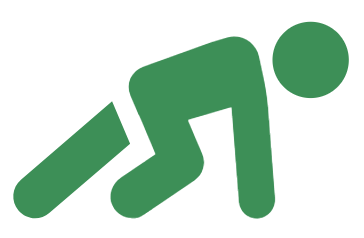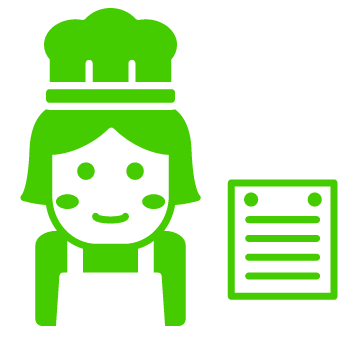# Neo4j Graph Algorithms

 Important - page not maintained This page is no longer being maintained and its content may be out of date. For the latest guidance, please visit the Neo4j Graph Data Science Library .

Graph algorithms provide one of the most potent approaches to analyzing connected data because their mathematical calculations are specifically built to operate on relationships. They describe steps to be taken to process a graph to discover its general qualities or specific quantities.

## Neo4j Graph Data Science Library (GDSL)

Neo4j Graph Data Science is a library that provides efficiently implemented, parallel versions of common graph algorithms for Neo4j 3.x and Neo4j 4.x exposed as Cypher procedures. It forms the core part of your Graph Data Science platform.

Amy Hodler and Alicia Frame also explain more about the library and share hands on examples in this talk from the Connections: Graph Data Science event.

The library contains implementations for the following types of algorithms:

• Path Finding - these algorithms help find the shortest path or evaluate the availability and quality of routes

• Centrality - these algorithms determine the importance of distinct nodes in a network

• Community Detection - these algorithms evaluate how a group is clustered or partitioned, as well as its tendency to strengthen or break apart

• Similarity - these algorithms help calculate the similarity of nodes

• Topological link prediction - these algorithms determine the closeness of pairs of nodes

• Node Embeddings - these algorithms compute vector representations of nodes in a graph.

• Node Classification - this algorithm uses machine learning to predict the classification of nodes.

• Link prediction - these algorithms use machine learning to predict new links between pairs of nodes

## Getting StartedThere are several ways to get started with graph algorithms:

Sandbox

No download required. Start using Neo4j Graph Algorithms within seconds through a built-in guide and dataset.

NEuler Graph Data Science Playground

No-code graph algorithms using this Graph App that provides a UI on top of the Graph Data Science Library.

Free online training

Learn how to use graph algorithms hands-on in the Data Science and Applied Graph Algorithms courses

## TutorialsThe following guides provide hands on examples of the different algorithms in the Graph Data Science Library.

## How-To GuidesThe recipes show how to use the Graph Data Science Library to solve common problems.

## ConceptsThe following guides provide more details and background for parts of the Graph Data Science Library and related topics.